voltage of only 1.5 volts, more than one cell is needed. To obtain the higher voltage, the cells are connected in series as shown in figure 2-6."> Series-Connected CellsCustom Search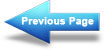Other Types of Cells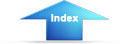Matter, Energy, and ElectricityBattery ConstructionSeries-Connected Cells

Assume that a load requires a power supply of 6 volts and a current capacity of 1/8 ampere. Since a single cell normally supplies a voltage of only 1.5 volts, more than one cell is needed. To obtain the higher voltage, the cells are connected in series as shown in figure 2-6.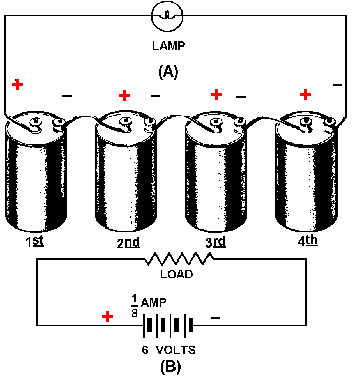Figure 2-6. - (A) Pictorial view of series-connected cells; (B) Schematic of series connection.

Figure 2-6 view B is a schematic representation of the circuit shown in figure 2-6 view A. The load is shown by the resistance symbol and the battery is indicated by one long and one short line per cell.

In a series hookup, the negative electrode (cathode) of the first cell is connected to the positive electrode (anode) of the second cell, the negative electrode of the second to the positive of the third, etc. The positive electrode of the first cell and negative electrode of the last cell then serve as the terminals of the battery. In this way, the voltage is 1.5 volts for each cell in the series line. There are four cells, so the output terminal voltage is 1.5 x 4, or 6 volts. When connected to the load, 1/8 ampere flows through the load and each cell of the battery. This is within the capacity of each cell. Therefore, only four series-connected cells are needed to supply this particular load.

CAUTION

When connecting cells in series, connect alternate terminals together (- to +, - to +, etc.) Always have two remaining terminals that are used for connection to the load only. Do not connect the two remaining terminals together as this is a short across the battery and would not only quickly discharge the cells but could cause some types of cells to explode.

Parallel-Connected Cells

In this case, assume an electrical load requires only 1.5 volts, but will require 1/2 ampere of current. (Assume that a single cell will supply only 1/8 ampere.) To meet this requirement, the cells are connected in parallel, as shown in figure 2-7 view Aand schematically represented in 2-7 view B. In a parallel connection, all positive cell electrodes are connected to one line, and all negative electrodes are connected to the other. No more than one cell is connected between the lines at any one point; so the voltage between the lines is the same as that of one cell, or 1.5 volts. However, each cell may contribute its maximum allowable current of 1/8 ampere to the line. There are four cells, so the total line current is 1/8 x 4, or 1/2 ampere. In this case four cells in parallel have enough capacity to supply a load requiring 1/2 ampere at 1.5 volts.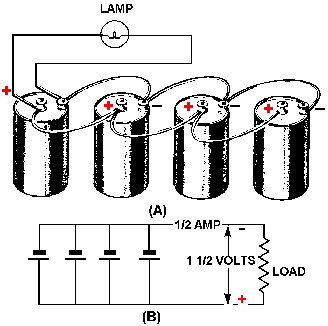Figure 2-7. - (A) Pictorial view of parallel-connected cells; (B) Schematic of parallel connection.

Series-Parallel-Connected Cells

Figure 2-8 depicts a battery network supplying power to a load requiring both a voltage and a current greater than one cell can provide. To provide the required 4.5 volts, groups of three 1.5-volt cells are connected in series. To provide the required 1/2 ampere of current, four series groups are connected in parallel, each supplying 1/8 ampere of current.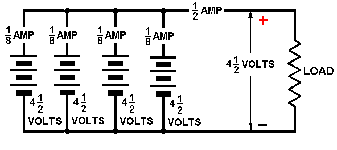Figure 2-8. - Schematic of series-parallel connected cells.

The connections shown have been used to illustrate the various methods of combining cells to form a battery. Series, parallel, and series-parallel circuits will be covered in detail in the next chapter, "Direct Current."

Some batteries are made from primary cells. When a primary-cell battery is completely discharged, the entire battery must be replaced. Because there is nothing else that can be done to primary cell batteries, the rest of the discussion on batteries will be concerned with batteries made of secondary cells.

Q25.What does the term battery normally refer to?   Test Yourself
Q26.What are the three ways of combining cells, and what is each used for?   Test YourselfIntegrated Publishing, Inc. - A (SDVOSB) Service Disabled Veteran Owned Small Business